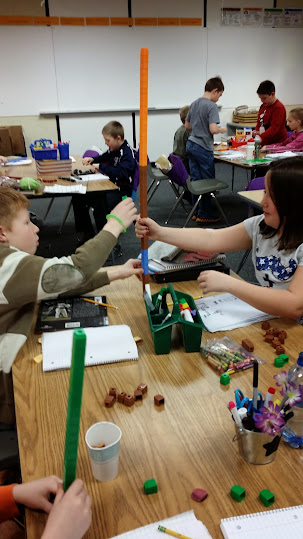# All Hands In for Improper Fractions

48 teachers like this lesson
Print Lesson

## Objective

SWBAT convert mixed numbers to improper fractions using unifix cubes.

#### Big Idea

In this lesson, students explore the relationship between mixed numbers and improper fractions with a hands on activity.

## Number Hook

5 minutes

To engage my students in thinking about numbers today I have a number trick for them I call Birthday Magic.

This trick works every time - as long as the math is correct- and students love it when I correctly guess their birthdays.

First tell students to multiply the month of their birthday by 5.  (January = 1, February = 2, March = 3 etc.)

Next, add 6 to that number.

Then multiply the new answer by 4.

Now, add 9 to this product.

Next, multiply the new sum by 5.

Next, I have my students then add their date of the birthday to this answer. (Just their month date, not year.  For example, someone born September 26 would simply add 26 during this step.)

Now, I ask a student to tell me their last answer.  To this number I subtract 165.  The last two numbers of this answer is the students birthday.  The first one or two numbers will be the month and the last one or two numbers will be the day.

## Warm Up

5 minutes

For this warm up, I present students with this review problem.

Vanessa grows sunflowers over the summer. She has 8 sunflowers and each sunflower measures 9/12 of a foot when they are first put into the garden. If she were to lay them end to end, how long would her 8 sunflowers measure

Note:  Most students were able to see that they could calculate 8 x 9/12 and get 72/12 which is equal to 6 feet or 72 inches.  Other students saw that 9/12 was the same as 9 inches and then multiplied 9 inches 8 times to also get 72 inches.  A few students used repeated addition.

## Concept Development

45 minutes

This lesson provides a hands-on approach to converting mixed numbers to improper fractions. Students use cubes as manipulatives while exploring the relationship between improper fractions and mixed numbers.  .

I begin this lesson by grouping students in pairs. I provide each group with unifex cubes and tell students that in today's lesson they will convert improper fractions to mixed numbers. I remind students that  an improper fraction is a fraction with a numerator that is greater than its denominator (ex:), and a mixed number is a number that includes both a whole number and a fraction ().

Before I have students use the cubes as a math tool, I give them  3 minutes to build or "play" with the cubes on their own. I have found that this time is so important in order for my students to then use the cubes as tools. Without this exploration time, students want to build and play during our math time.  By giving them a few short minutes in the beginning, they are much more willing to use the cubes as tools later.

You can see in this photo student utilizing their time to build a cube tower.After the three minutes, I direct students to model a mixed number like 2 2/3 with cubes.

My students are used to drawing area models to model fractions and have had practice doing this prior to this lesson.

Next I use direct instruction and I say to students, “I am going to divide these eight parts into groups of three.” I ask students to explain why the eight counters need to be placed into groups of three . [The denominator is three, so a whole is made up of three parts.]

Then I demonstrate moving the eight counters into groups of three. I have students do the same with their counters. Next I say to students, “Instead of eight individual parts, I now have two groups of three with two counters left over. How many wholes do we have?” [2.] “How do you know?” [There are two groups of counters that each represents a whole, each group has three parts in a set of three, 3/3].

I then ask students how many individual pieces are left. [2.] I ask students what part of the whole these two pieces represents. [2/3].

Next, on the board I write  3/3 + 3/3 + 2/3.

I then explain that we can add the thirds together to find that  2 2/3 is equal to 8/3.

Next, I display several problems for students to model, converting mixed numbers to improper fractions.

As students work, I check for understanding.  I ask students to share the steps needed to convert the mixed number to an improper fraction.

Listen in as this student is converting a mixed number to an improper fraction. She has discovered a shortcut of multiplying, but got a little confused.  I ask her several clarifying questions to get her back on track.

At the end of the lesson, students explain how to convert mixed numbers to improper fractions. I listen for terms like numeratordenominatorwholepartimproper fraction, and mixed number

Note:  Many students, by the end of this lesson, have discovered shortcuts for converting mixed numbers to improper fractions.  I spend about 5 - 7 minutes showing some of the shortcuts other students have discovered and help students see the connections between using the manipulatives and why the algorithm of multiplying the wholes with the denominator and adding the numerator works.  This is usually an exciting time in my class as students own the knowledge and make sense of the algorithm.  They delight in understanding why the "trick" their parents may have taught them works.